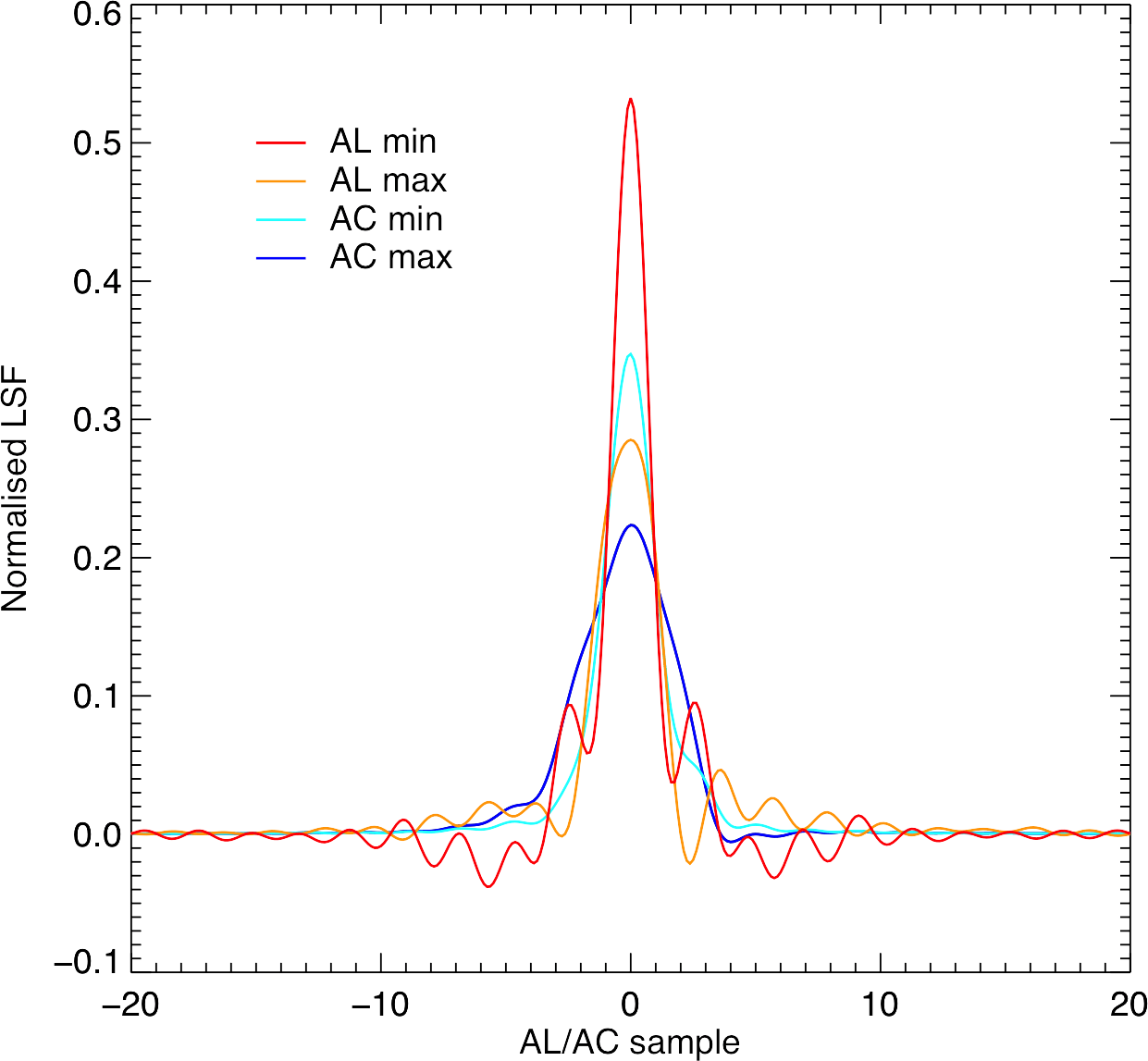# 6.3.3 Wavelength calibration

The most important calibration necessary for estimating $V_{\rm rad}$ is the wavelength calibration. It is implemented in the pipeline which calculates the associated calibration parameters and their spatial and temporal dependence. The basic principle of the wavelength calibration is that the wavelength associated with a sample can be expressed as a function of the FoV coordinates $(\eta,\zeta)$ of the source at the time when the sample crosses the CCD fiducial line (i.e. AL pixel 2253.5).

A self-calibration approach is adopted and some of the RVS spectra are used as calibrators (Figure 6.6 shows an example of these). The pipeline detects the spectral lines in the calibrator spectra, identifies them and assigns a rest wavelength by comparison with a synthetic spectrum template. The dispersion function used is:

 $\lambda_{\rm observed}=\lambda_{\rm rest}\left(1+\frac{V(s)}{c}\right)=C_{00}+% C_{10}\eta+C_{20}\eta^{2}+C_{01}\zeta+C_{11}\eta\zeta$ (6.1)

where:

• $\lambda_{\rm observed}$ is the wavelength associated with the centre of the sample;

• $C_{mn}$ are the unknown calibration coefficients;

• $V(s)$ is the unknown Gaia-centric radial velocity of the calibration star;

• $\eta$ and $\zeta$ are the field angles FoV coordinates of the calibration stars at the fiducial time of the CCD.

To avoid the degeneracy due to the fact that a shift in the dispersion law can be compensated by a shift in the radial velocity $V(s)$ of the calibration stars, the auxiliary standard stars (Section 6.2.3) are used to fix the zero-point. More details of how Equation 6.1 and the wavelength calibration is implemented are in Sartoretti et al. (2018, Section 5.3). The systematic shifts that were present in the DR2 data between the two FoVs (in the opposite sense) and between the different rows are now reduced thanks to the use of the calibrated LSF-AL (Section 6.3.4) to generate the templates.

The wavelength calibration process is repeated for all the calibration units for all CCDs and FoV of a trending epoch. Once the coefficients for the entire trending epoch are obtained, a trending module fits a curve to describe the emerging trend for each coefficient. The trending functions are used to calibrate the spectra acquired at any time within the trending epoch, and at any position (see Figure 6.12 for examples of trending functions).Figure 6.4: Comparison of AL and AC LSFs. These are constructed using fixed basis functions (Hn⁢(u)) and trended amplitudes (hn), described in Section 6.3.4. CaU LSFs are normalised to have an area under the curve equal to one. Trended LSFs have an area under their curves very close to unity already, so they are not re-normalised. The max and min refer to maximum and minimum FWHM from trend epoch 3, FoV 1 (preceding), Row 4, Strip 16. The minimum AL FWHM occurs at the best focus within epoch 3 (OBMT 1527.5), after payload decontamination number 4 (OBMT 1317.3), whereas the maximum AL FWHM occurs at the worst focus within epoch 3 (OBMT 2279.5), before payload decontamination number 5 (OBMT 2330.8): see Table 1.11 and Figure 1.12 for more details. An illustrative maximum AC FWHM occurs at OBMT 1444.5 and the next minimum AC FWHM occurs at OBMT 1444.75. The difference of 0.25 revolutions is explained by the AC smearing effect, induced by the combination of the transversal motion (due to precession) and the optical distortions. Figure by Mike Smith.